Question

# engineering strain：0.0054 engineering sress：62.05741608 true strain：0.005385472 true stress：62.39252612 how to calculate LOG true stress vs LOG...

engineering strain：0.0054
engineering sress：62.05741608
true strain：0.005385472
true stress：62.39252612

how to calculate LOG true stress vs LOG true strain
calculate strain hardening factor n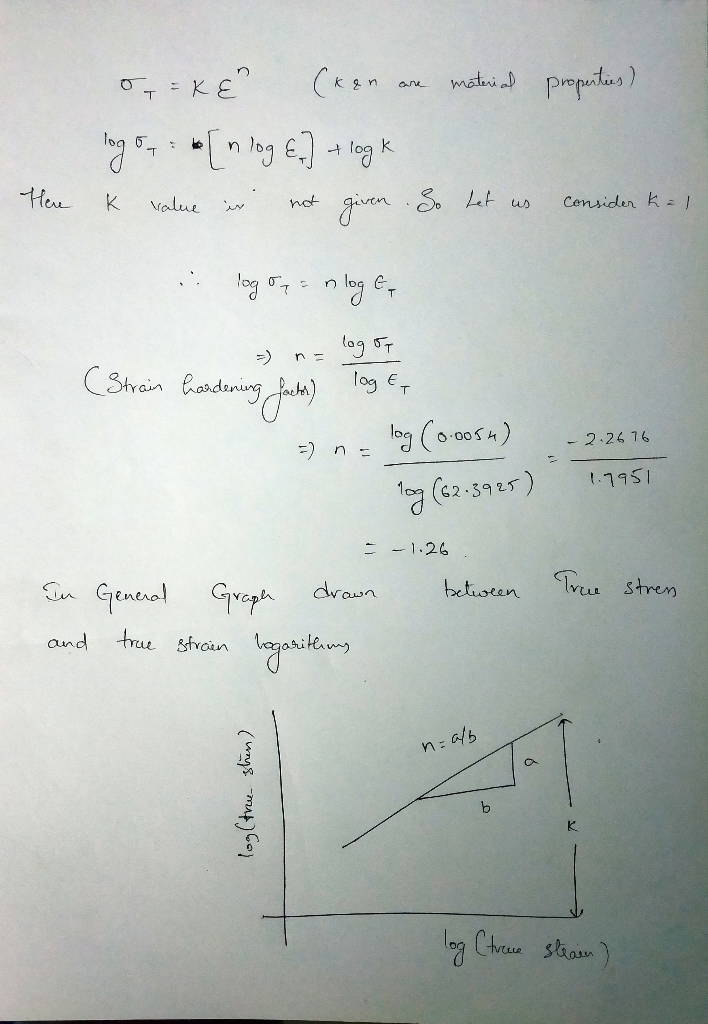If certain number values of true stress are there then we can calculate from the Graph by drawing a tangent to the the curve formed.

Number of values of stress will occur when cross sectional areas near the failure are taken a 3-5 readings

#### Earn Coins

Coins can be redeemed for fabulous gifts.

Similar Homework Help Questions
• ### 2. If an engineering stress-strain curve shows a stress of 130ksi at a strain of 1890...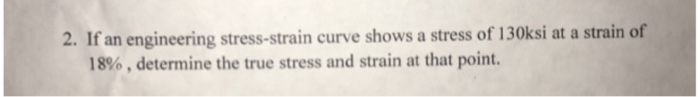2. If an engineering stress-strain curve shows a stress of 130ksi at a strain of 1890 , determine the true stress and strain at that point.

• ### HotLW Problem: Giving the engineering stress - engineering strain carve for an aluminum alloy, Calalate: 400FTTTTTTTTTT...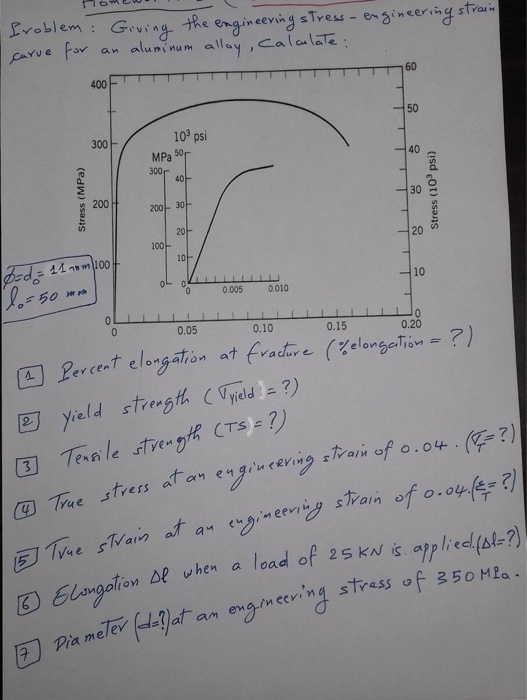HotLW Problem: Giving the engineering stress - engineering strain carve for an aluminum alloy, Calalate: 400FTTTTTTTTTT 10 psi 300 MPa 50 \$ Stress (MPa) Stress (10 psi) 8 100- 10/ bd = 110m 1oof lo=50 mm 0 0 0.005 0.010 o 0.05 0. 100 . 150 .20 6 percent elongation at fracture (% elongation = ?) 2 yield strength (Tyield = ?) 3 Tensile strength (TS = ?) ♡ True stress at an engineering strain of 0.04. (I=?) 5 True...

• ### 1. The following is the true stress/true strain curve for a medium carbon steel. Based on...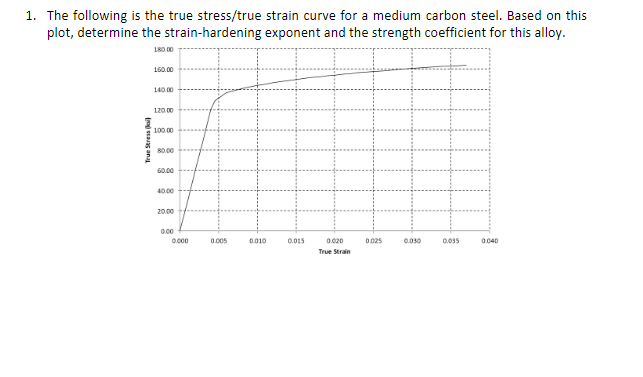1. The following is the true stress/true strain curve for a medium carbon steel. Based on this plot, determine the strain-hardening exponent and the strength coefficient for this alloy 180 00T 160.00 14000-4- 12000 10000 6000 40.00 20.00 C000.005 0010 .01500200025 0.030 0035 040 True Strain

• ### 2) Draw two schematic graphs using pencil showing a typical stress-strain curve for aluminum. The first...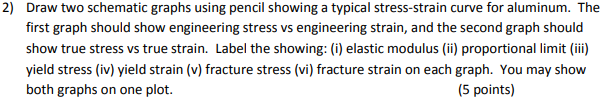2) Draw two schematic graphs using pencil showing a typical stress-strain curve for aluminum. The first graph should show engineering stress vs engineering strain, and the second graph should show true stress vs true strain. Label the showing: (i) elastic modulus (ii) proportional limit (iii) yield stress (iv) yield strain (v) fracture stress (vi) fracture strain on each graph. You may show both graphs on one plot. (5 points)

• ### For some metal alloy, a true stress of 345 MPa (50,000 psi) produces a plastic true...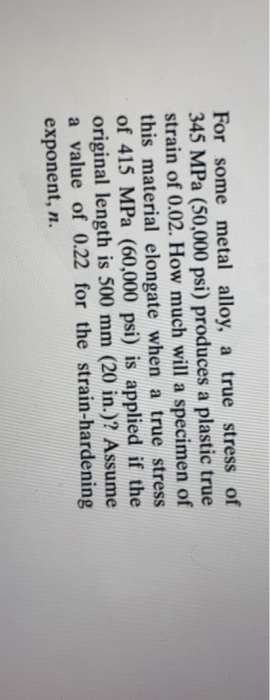For some metal alloy, a true stress of 345 MPa (50,000 psi) produces a plastic true strain of 0.02. How much will a specimen of this material elongate when a true stress of 415 MPa (60,000 psi) is applied if the original length is 500 mm (20 in.)? Assume a value of 0.22 for the strain-hardening exponent, n.

• ### 11 GIVEN K=299 MPA n=0.33 hardening exponent If the true stress is half of K in...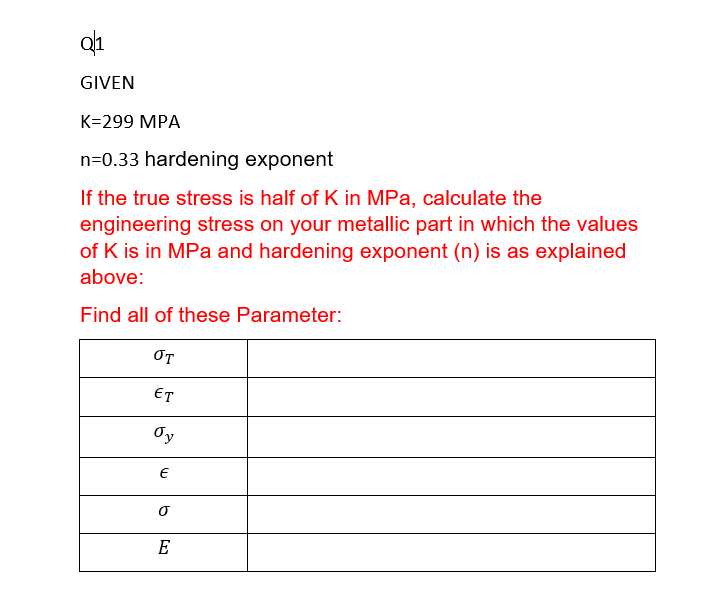11 GIVEN K=299 MPA n=0.33 hardening exponent If the true stress is half of K in MPa, calculate the engineering stress on your metallic part in which the values of K is in MPa and hardening exponent (n) is as explained above: Find all of these Parameter: OT ET Oy E o E

• ### Problem 9) a) b) What is the difference between the engineering stress and true stress Explain...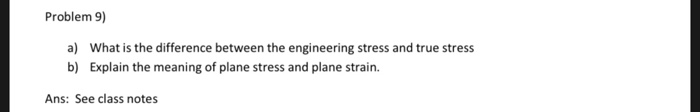Problem 9) a) b) What is the difference between the engineering stress and true stress Explain the meaning of plane stress and plane strain. Ans: See class notes

• ### copper, the tnae stress and tue strain are relatod by the oy 530 where irue stress...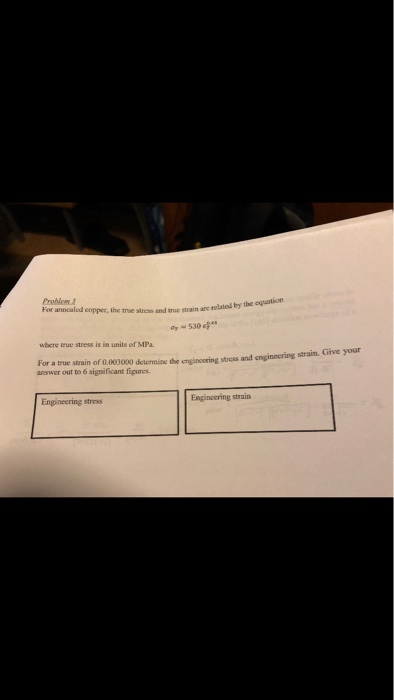copper, the tnae stress and tue strain are relatod by the oy 530 where irue stress is in units of MPa For a true strain of 0.003000 deitermine the engincering stress and engineering strain. Give your answer out to 6 significant figures Engineering stress Engincering strain

• ### 3. A set of tensile test data is provided. The first column is the force measurement...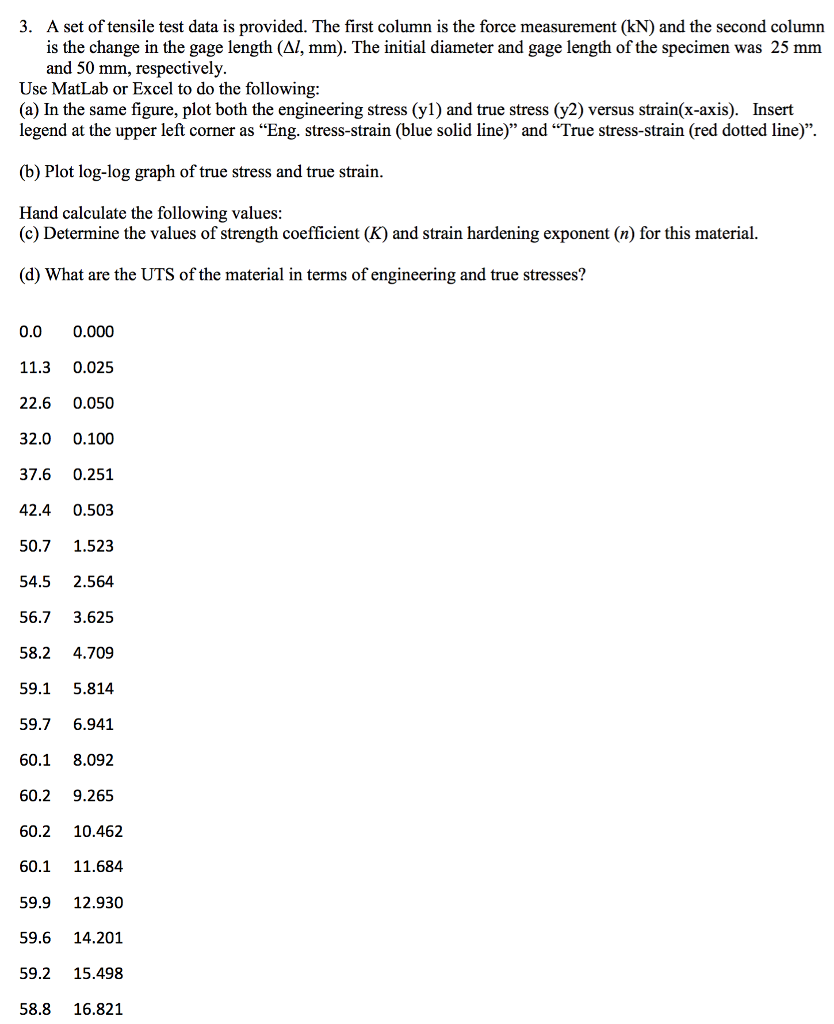3. A set of tensile test data is provided. The first column is the force measurement (kN) and the second column is the change in the gage length (Al, mm). The initial diameter and gage length of the specimen was 25 mm and 50 mm, respectively Use MatLab or Excel to do the following (a) In the same figure, plot both the engineering stress (y1) and true stress (y2) versus strain(x-axis). Insert legend at the upper left corner as "Eng....

• ### 6) llustrate (qualitatively) how you would expect the engineering stress-strain curve of a rate- sensitive metal...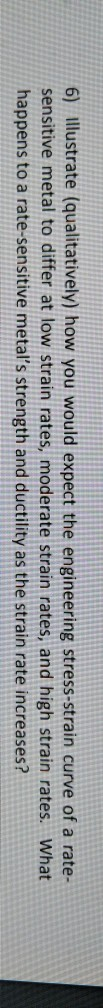6) llustrate (qualitatively) how you would expect the engineering stress-strain curve of a rate- sensitive metal to differ at low strain rates, moderate strain rates, and high strain rates. What happens to a rate-sensitive metal's strength and ductility as the strain rate increases?